## 三角剖分表示法

### 二维和三维域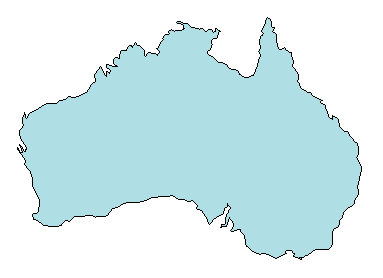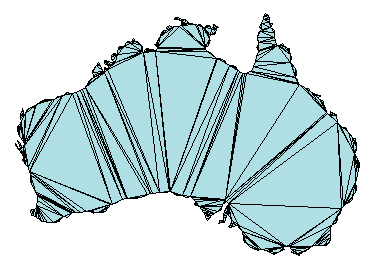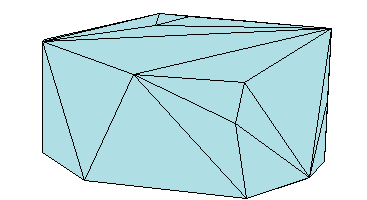### 三角剖分矩阵格式

MATLAB® 使用矩阵格式表示三角剖分。此格式包含以下两个部分：

• 采用一个矩阵表示的顶点，该矩阵中每行包含三角剖分中一个点的坐标。

• 采用一个矩阵表示的三角剖分连接，该矩阵中每行定义一个三角形或四面体。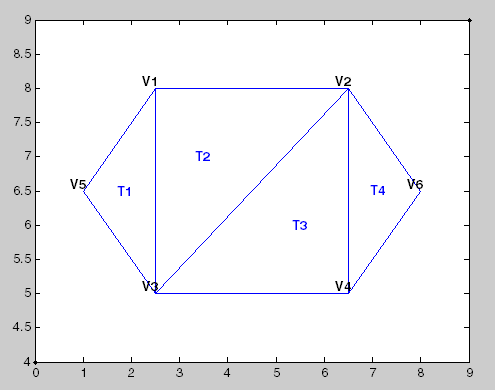V12.58.0
V26.58.0
V32.55.0
V46.55.0
V51.06.5
V68.06.5

T1531
T2321
T3342
T4462

• 二维三角剖分，包含由顶点和边线限定的三角形

• 三维曲面三角剖分，包含由顶点和边线限定的三角形

• 三维三角剖分，包含由顶点、边线和面限定的四面体

### 使用三角剖分类查询三角剖分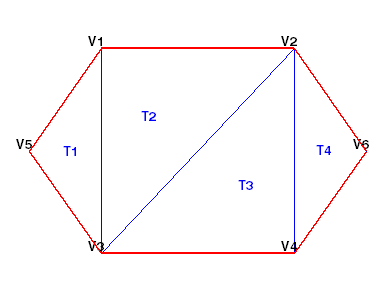#### 三角剖分类

• 将矩阵格式的现有数据传递到 `triangulation`。这些数据可以是 MATLAB 函数的输出，例如 `delaunay``convhull`。您可以导入通过其他软件应用程序创建的三角剖分数据。使用导入的数据时，请确保连接数据使用从 1 开始而不是从 0 开始的索引来引用顶点数组。

• 将一组点传递到 `delaunayTriangulation`。生成的 Delaunay 三角剖分一种特殊的 `triangulation`。这意味着可以对数据执行任何 `triangulation` 查询以及任何 Delaunay 特定的查询。在比较正式的 MATLAB 语言术语中，`delaunayTriangulation``triangulation` 的子类。

```P = [ 2.5 8.0 6.5 8.0 2.5 5.0 6.5 5.0 1.0 6.5 8.0 6.5];```

```T = [5 3 1; 3 2 1; 3 4 2; 4 6 2];```

`TR = triangulation(T,P)`
```TR = triangulation with properties: Points: [6x2 double] ConnectivityList: [4x3 double] ```

`TR.Points`
```ans = 6×2 2.5000 8.0000 6.5000 8.0000 2.5000 5.0000 6.5000 5.0000 1.0000 6.5000 8.0000 6.5000 ```

`TR.ConnectivityList`
```ans = 4×3 5 3 1 3 2 1 3 4 2 4 6 2 ```

`Points``ConnectivityList` 属性定义三角剖分的矩阵数据。

`triangulation` 类是矩阵数据的封装类。真实益处是 `triangulation` 类方法的有用性。这些方法类似于接受 `triangulation` 和其他相关输入数据的函数。

`triangulation` 类提供了一种简单方法创建 `ConnectivityList` 属性矩阵的索引。访问三角剖分中的第一个三角形。

`TR.ConnectivityList(1,:)`
```ans = 1×3 5 3 1 ```

`TR(1,1)`
```ans = 5 ```

`TR(1,2)`
```ans = 3 ```

`TR(:,:)`
```ans = 4×3 5 3 1 3 2 1 3 4 2 4 6 2 ```

```figure triplot(TR) axis equal````boundaryedges = freeBoundary(TR)';`

```hold on plot(P(boundaryedges,1),P(boundaryedges,2),'-r','LineWidth',2) hold off``````P = [ 2.5 8.0 6.5 8.0 2.5 5.0 6.5 5.0 1.0 6.5 8.0 6.5]; DT = delaunayTriangulation(P)```
```DT = delaunayTriangulation with properties: Points: [6x2 double] ConnectivityList: [4x3 double] Constraints: [] ```

`DT(1,:)`
```ans = 1×3 5 3 1 ```

`DT(:,:)`
```ans = 4×3 5 3 1 3 4 1 1 4 2 4 6 2 ```

```triplot(DT) axis equal````IC = incenter(DT)`
```IC = 4×2 1.8787 6.5000 3.5000 6.0000 5.5000 7.0000 7.1213 6.5000 ```

```hold on numtri = size(DT,1); trilabels = arrayfun(@(P) {sprintf('T%d', P)}, (1:numtri)'); Htl = text(IC(:,1),IC(:,2),trilabels,'FontWeight','bold', ... 'HorizontalAlignment','center','Color','blue'); hold off``````P = [ 2.5 8.0 6.5 8.0 2.5 5.0 6.5 5.0 1.0 6.5 8.0 6.5]; T = delaunay(P); TR = triangulation(T,P); IC = incenter(TR);```

• `delaunayTriangulation` 类可以提供适合处理三角剖分的其他方法。例如，可以执行最近邻点和三角形内的点搜索。

• 它允许您编辑三角剖分以添加、移动或删除点。

• 它允许您创建约束性的 Delaunay 三角剖分。这允许您创建二维域的三角剖分。